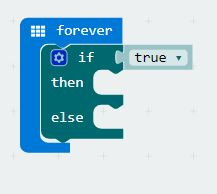GiggleBot - The Micro:Bit Robot
GiggleBot - The Micro:Bit Robot

Coding Examples — if then else

micro:bit Programming - Use the if then else statement in MakeCode

bbc microbit robot if then else if then else microbit robotIn this article we explore the "if then else" block in the MakeCode programming language.  This block is a logic block that extends the "if then" block.  We wrote an introduction to the "if then" block here.  In this example, we will show you how to program and if-then-else block and control a robot, helping the robot to decide if it should move forward or stop.